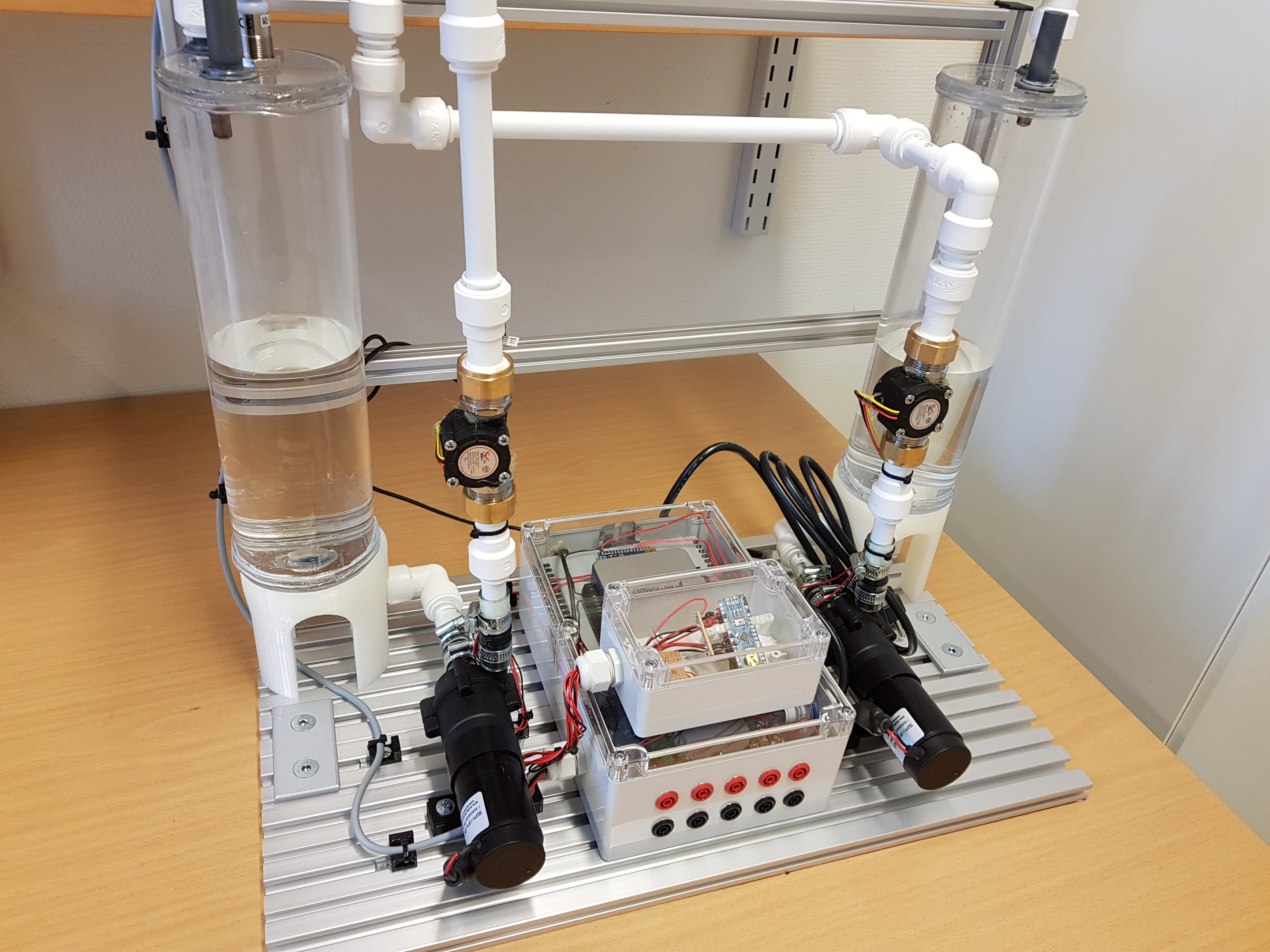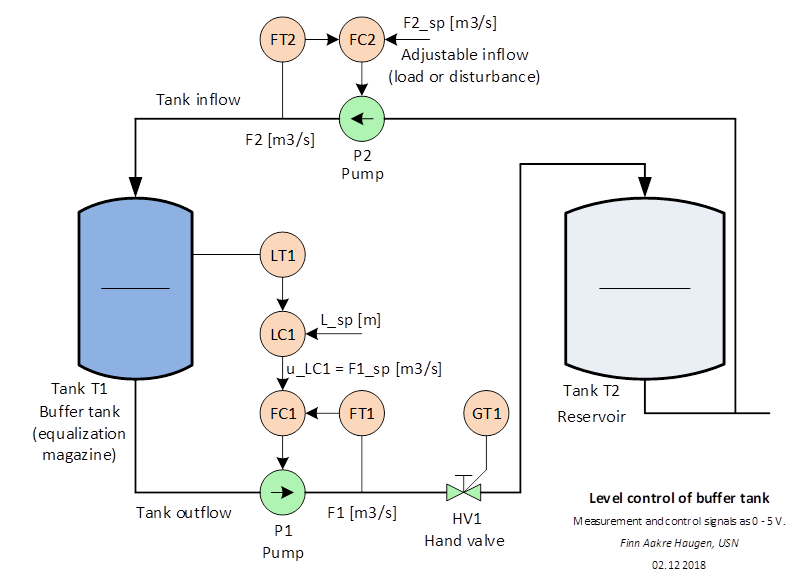# Lab Station: Buffer tank

University of South-Eastern Norway (campus Porsgrunn) has 10 laboratory buffer tanks, see Figure 1. The tanks are used in control courses in both bachelor and master programmes in technology.Figure 1: Buffer tank

## Technical description

Figure 2 shows a piping and instrumentation diagram (P&I D) of the tank including a level control system.Figure 2: Piping and instrumentation diagram (P&I D) of the buffer tank a level control system.

The measurement signals (analog inputs) from the sensors LT1, FT1 and FT2 are voltage signals in the range 0 5 V.

The control signals (analog outputs) to the actuators pump P1and pump P2 are voltage signals in the range 0 5 V.

A built-in NI USB-6001 IO device handles the IO. This IO device can be connected to a PC with a USB cable.

As an alternative to using the NI USB-6001 IO device, there are terminals on the rig for direct analog IO.

To appear.

To appear.

## Level controller

The control signal generated by the level controller is a demanded outflow:

u = F_out

The level controller may be a PI controller or some other controller.

## Mathematical model

Mass balance of the water in the tank leads to the following “volume balance” assuming water density is the same all over:

h’ = (F_in - F_out)/A

where:

·       h [cm] is the water level in the tank.

·       F_in [cm^3/s] is water inflow.

·       F_out [cm^3/s] is water outflow.

·       A [cm^2] is cross-sectional area of tank.

Nominal values and ranges:

·       h_nom = 15. Range: 0 - 30.

·       F_in_nom = 50. Range: 0 - 100.

·       F_out_nom = 50. Range: 0 - 100.

·       A = 56.7.